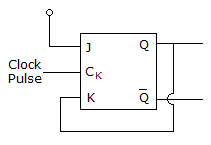# Electronics and Communication Engineering - Digital Electronics - Discussion

2.

In the given figure assume that initially Q = 1 with Clock Pulses being given, the subsequent states of Q will be[A]. 1, 0, 1, 0, 1...... [B]. 0, 0, 0, 0, 0...... [C]. 1, 1, 1, 1, 1...... [D]. 0, 1, 0, 1, 0......

Explanation:

No answer description available for this question.

 Prana said: (Mar 31, 2015) How please explain?

 Loknath Reddy D said: (Jul 30, 2015) J=? not given and for J=0 or 1 C is wrong answer.

 Guru said: (Oct 18, 2015) Qn+1 = JQ'+K'Q. Now put k = Q. Qn+1 = JQ'+1 = 1;

 Amar.B said: (Dec 16, 2015) If we assume j=0 and for the given condition answer should be 'B'?

 K Suman said: (Jan 4, 2016) Qn+1 = JQ'+K'Q. So observation Q = 1, K = Q substitute in that Qn+1 = J1'+Q'Q implies that Qn+1 = 0 again same repeats above Qn+1 = J. So answer not match.

 K Suman said: (Jan 4, 2016) None of the answer. We can't estimate the next because of, Qn+1 = JQ'+K'Q at clock 1. Q = 1, K = Q then Qn+1 = J(1)'+Q'Q == 0. At clock 2. Q = 0, K = 0 then from character equation. Output Qn+1 = J(0)'+(0)'0 == J so we can't estimate the next state.

 Bhanu said: (Jun 7, 2017) I think the Answer is D.

 Arnab Bhattacharya said: (Dec 7, 2017) If we assume that J is tied to +Vcc (logic 1) (assumption from the symbol), then K=1 makes the flipflop toggle thus making it Q=0. Next J=1, K=0 (since Q=0). So again Q becomes 1. This thing repeats and the output should be: 0,1,0,1... I think 'D' is the right option.

 Arnab Bhattacharya said: (Dec 7, 2017) If we assume, J is tied to Gnd/-ve (i.e. logic 0), then initial Q=1, makes K=1 thus resetting the flipflop making it output Q=0. Thus at next clock pulse Q=0, K=0, J=0 will hold the output making new output (Q=0). This will continue and the output will be: 0,0,0,0,0........ And Q' (inverted) will be: 1,1,1,1,1....... So, if J=0, The correct option will be 'B' I suppose.

 D Venkat said: (May 24, 2022) I think it should be option D.